Balancing Chemical Equations IPage 1

WATCH ALL SLIDES

Slide 1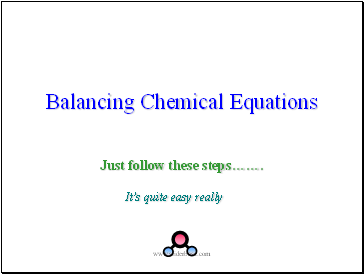Balancing Chemical Equations

Just follow these steps…….

It’s quite easy really

Slide 2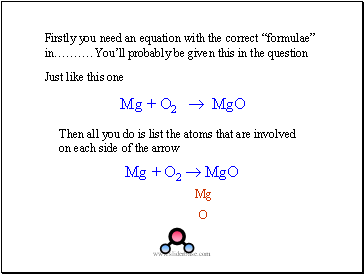Firstly you need an equation with the correct “formulae” in………. You’ll probably be given this in the question

Just like this one

Mg + O2  MgO

Mg

O

Slide 3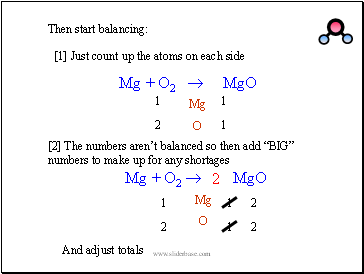Just count up the atoms on each side

Then start balancing:

1

1

1

2

 The numbers aren’t balanced so then add “BIG” numbers to make up for any shortages

2

2

2

Slide 4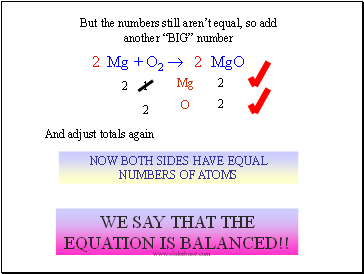But the numbers still aren’t equal, so add another “BIG” number

2

And adjust totals again

NOW BOTH SIDES HAVE EQUAL NUMBERS OF ATOMS

WE SAY THAT THE EQUATION IS BALANCED!!

2

Slide 5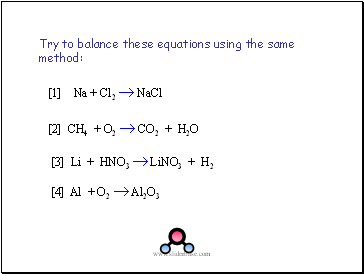Try to balance these equations using the same method:

 Na + Cl2  NaCl

 CH4 + O2  CO2 + H2O

 Al + O2  Al2O3

 Li + HNO3  LiNO3 + H2

Slide 6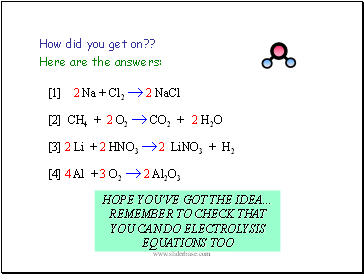How did you get on??

 2 Na + Cl2  2 NaCl

 CH4 + 2 O2  CO2 + 2 H2O

 4 Al + 3 O2  2 Al2O3

 2 Li + 2 HNO3  2 LiNO3 + H2

Here are the answers:

HOPE YOU’VE GOT THE IDEA… REMEMBER TO CHECK THAT YOU CAN DO ELECTROLYSIS EQUATIONS TOO

Slide 7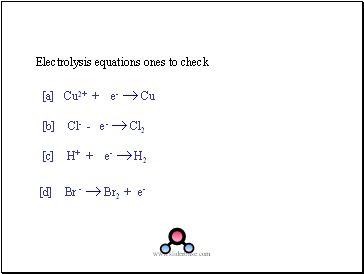Electrolysis equations ones to check

[a] Cu2+ + e-  Cu

[b] Cl- - e-  Cl2

[c] H+ + e-  H2

[d] Br -  Br2 + e-

Slide 8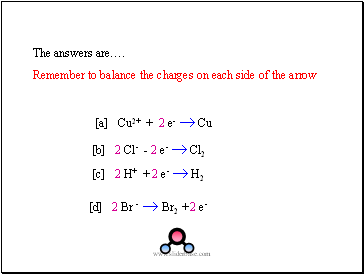Remember to balance the charges on each side of the arrow

[a] Cu2+ + 2 e-  Cu

[b] 2 Cl- - 2 e-  Cl2

[c] 2 H+ + 2 e-  H2

[d] 2 Br -  Br2 + 2 e-

Slide 9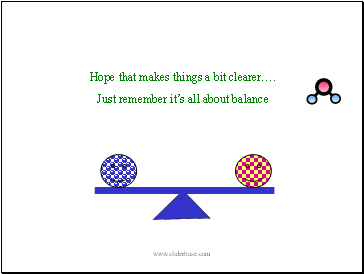Hope that makes things a bit clearer….

Just remember it’s all about balance